# matplotlib.pyplot.contour¶

`matplotlib.pyplot.``contour`(*args, **kwargs)[source]

Plot contours.

Call signature:

```contour([X, Y,] Z, [levels], **kwargs)
```

`contour()` and `contourf()` draw contour lines and filled contours, respectively. Except as noted, function signatures and return values are the same for both versions.

Parameters: X, Y : array-like, optional The coordinates of the values in Z. X and Y must both be 2-D with the same shape as Z (e.g. created via `numpy.meshgrid()`), or they must both be 1-D such that `len(X) == M` is the number of columns in Z and `len(Y) == N` is the number of rows in Z. If not given, they are assumed to be integer indices, i.e. `X = range(M)`, `Y = range(N)`. Z : array-like(N, M) The height values over which the contour is drawn. levels : int or array-like, optional Determines the number and positions of the contour lines / regions. If an int n, use n data intervals; i.e. draw n+1 contour lines. The level heights are automatically chosen. If array-like, draw contour lines at the specified levels. The values must be in increasing order. :class:`~matplotlib.contour.QuadContourSet` corner_mask : bool, optional Enable/disable corner masking, which only has an effect if Z is a masked array. If `False`, any quad touching a masked point is masked out. If `True`, only the triangular corners of quads nearest those points are always masked out, other triangular corners comprising three unmasked points are contoured as usual. Defaults to `rcParams['contour.corner_mask']`, which defaults to `True`. colors : color string or sequence of colors, optional The colors of the levels, i.e. the lines for `contour` and the areas for `contourf`. The sequence is cycled for the levels in ascending order. If the sequence is shorter than the number of levels, it's repeated. As a shortcut, single color strings may be used in place of one-element lists, i.e. `'red'` instead of `['red']` to color all levels with the same color. This shortcut does only work for color strings, not for other ways of specifying colors. By default (value None), the colormap specified by cmap will be used. alpha : float, optional The alpha blending value, between 0 (transparent) and 1 (opaque). cmap : str or `Colormap`, optional A `Colormap` instance or registered colormap name. The colormap maps the level values to colors. Defaults to `rcParams["image.cmap"]`. If given, colors take precedence over cmap. norm : `Normalize`, optional If a colormap is used, the `Normalize` instance scales the level values to the canonical colormap range [0, 1] for mapping to colors. If not given, the default linear scaling is used. vmin, vmax : float, optional If not None, either or both of these values will be supplied to the `Normalize` instance, overriding the default color scaling based on levels. origin : {None, 'upper', 'lower', 'image'}, optional Determines the orientation and exact position of Z by specifying the position of `Z[0, 0]`. This is only relevant, if X, Y are not given. None: `Z[0, 0]` is at X=0, Y=0 in the lower left corner. 'lower': `Z[0, 0]` is at X=0.5, Y=0.5 in the lower left corner. 'upper': `Z[0, 0]` is at X=N+0.5, Y=0.5 in the upper left corner. 'image': Use the value from `rcParams["image.origin"]`. Note: The value None in the rcParam is currently handled as 'lower'. extent : (x0, x1, y0, y1), optional If origin is not None, then extent is interpreted as in `matplotlib.pyplot.imshow()`: it gives the outer pixel boundaries. In this case, the position of Z[0,0] is the center of the pixel, not a corner. If origin is None, then (x0, y0) is the position of Z[0,0], and (x1, y1) is the position of Z[-1,-1]. This keyword is not active if X and Y are specified in the call to contour. locator : ticker.Locator subclass, optional The locator is used to determine the contour levels if they are not given explicitly via levels. Defaults to `MaxNLocator`. extend : {'neither', 'both', 'min', 'max'}, optional Unless this is 'neither', contour levels are automatically added to one or both ends of the range so that all data are included. These added ranges are then mapped to the special colormap values which default to the ends of the colormap range, but can be set via `matplotlib.colors.Colormap.set_under()` and `matplotlib.colors.Colormap.set_over()` methods. xunits, yunits : registered units, optional Override axis units by specifying an instance of a `matplotlib.units.ConversionInterface`. antialiased : bool, optinal Enable antialiasing, overriding the defaults. For filled contours, the default is True. For line contours, it is taken from `rcParams["lines.antialiased"]`. Nchunk : int >= 0, optional If 0, no subdivision of the domain. Specify a positive integer to divide the domain into subdomains of nchunk by nchunk quads. Chunking reduces the maximum length of polygons generated by the contouring algorithm which reduces the rendering workload passed on to the backend and also requires slightly less RAM. It can however introduce rendering artifacts at chunk boundaries depending on the backend, the antialiased flag and value of alpha. linewidths : float or sequence of float, optional Only applies to `contour`. The line width of the contour lines. If a number, all levels will be plotted with this linewidth. If a sequence, the levels in ascending order will be plotted with the linewidths in the order specified. Defaults to `rcParams["lines.linewidth"]`. linestyles : {None, 'solid', 'dashed', 'dashdot', 'dotted'}, optional Only applies to `contour`. If linestyles is None, the default is 'solid' unless the lines are monochrome. In that case, negative contours will take their linestyle from `rcParams["contour.negative_linestyle"]` setting. linestyles can also be an iterable of the above strings specifying a set of linestyles to be used. If this iterable is shorter than the number of contour levels it will be repeated as necessary. hatches : List[str], optional Only applies to `contourf`. A list of cross hatch patterns to use on the filled areas. If None, no hatching will be added to the contour. Hatching is supported in the PostScript, PDF, SVG and Agg backends only.

Notes

1. `contourf()` differs from the MATLAB version in that it does not draw the polygon edges. To draw edges, add line contours with calls to `contour()`.

2. contourf fills intervals that are closed at the top; that is, for boundaries z1 and z2, the filled region is:

```z1 < Z <= z2
```

There is one exception: if the lowest boundary coincides with the minimum value of the Z array, then that minimum value will be included in the lowest interval.

## Examples using `matplotlib.pyplot.contour`¶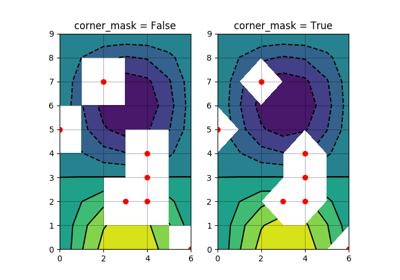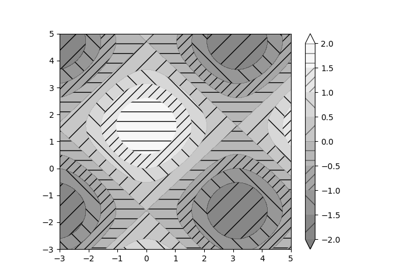Contourf Hatching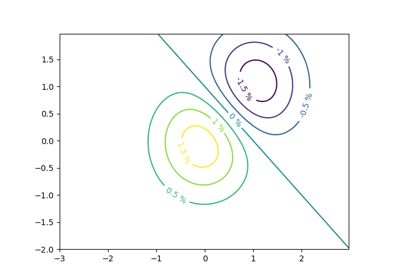Contour Label Demo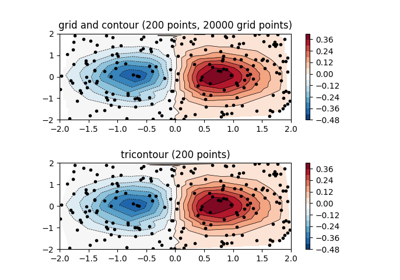Contour plot of irregularly spaced data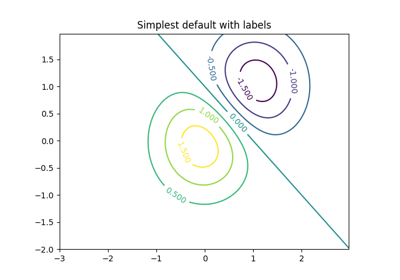Contour Demo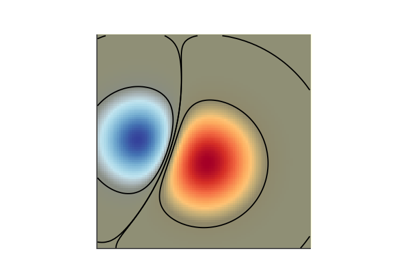Blend transparency with color in 2-D images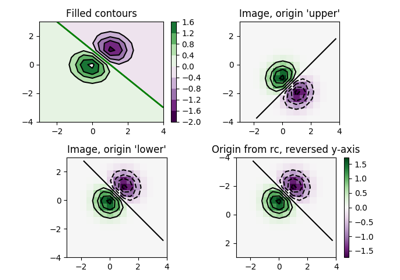Contour Image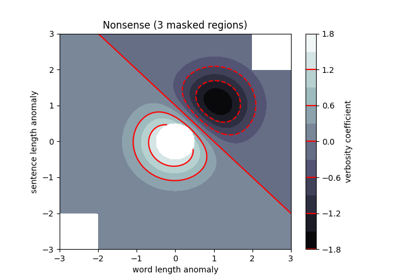Contourf Demo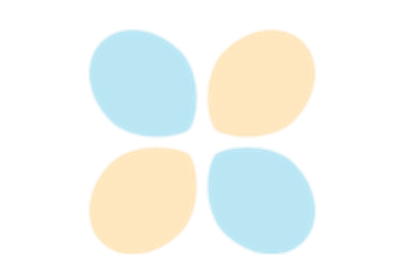Interactive functions